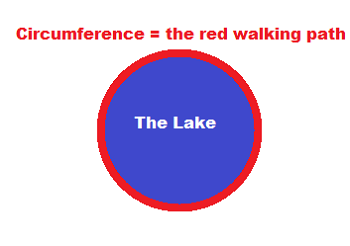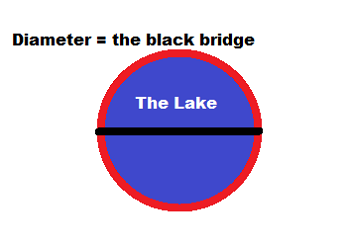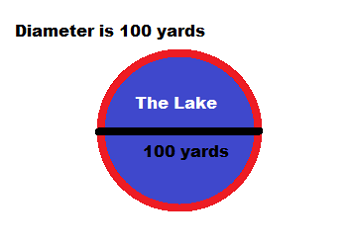# Circumference Lesson for Kids: Definition & Formula

Instructor: Bethany Calderwood

Bethany has taught special education in grades PK-5 and has a master's degree in special education.

In this lesson, you will learn what circumference is, its formula and the tools you need to find circumference. Then, you can try finding the circumference of a circle on your own!

## Circumference: A Walk Around

Imagine you are going for a walk around a lake, and you want to know how far you went. How would you measure the distance around the lake? The walking path around the lake is a circle. To find the distance of the walking path, you need to find the circumference of the circle. Circumference is the distance around the outside of a circle.## The Formula

So how do we calculate the circumference of our lake? Here is the formula:

Circumference = pi x diameter

So, there are two tools me must have in order to find circumference: diameter and pi. Let's discuss these further.

### Diameter: The First Tool

Diameter is a straight line that goes from one side of the circle to the other and passes through the center of the circle. A line across the circle is a diameter only if it crosses the circle's center.

Look at the lake again. See the bridge that goes across the middle of the circle? Because the bridge starts on one side of the path, goes over the center of the circle, and ends at the path on the other side of the circle, that bridge is the diameter of the lake path.### Pi: The Second Tool

Pi is a number that is often used in math. It is an infinite number equal to approximately 22 divided by 7, and it's usually rounded to 3.14.

## Try It Yourself: An Exercise

Now that you know formula and tools for circumference, let's find the distance around the lake.#### What We Know

• The bridge across the lake is the diameter of the circle.
• The bridge is 100 yards long, so 100 yards is the diameter of the circle.
• Circumference = pi x diameter

#### Put the Numbers Into the Formula

• Replace 'pi' with 3.14 and 'diameter' with 100 yards:
• Circumference = 3.14 x 100 yards

#### Do the Math

• 3.14 x 100 yards = 314 yards
• Circumference = 314 yards

Your walk around the lake is 314 yards.

To unlock this lesson you must be a Study.com Member.

### Register to view this lesson

Are you a student or a teacher?

#### See for yourself why 30 million people use Study.com

##### Become a Study.com member and start learning now.
Back
What teachers are saying about Study.com

### Earning College Credit

Did you know… We have over 200 college courses that prepare you to earn credit by exam that is accepted by over 1,500 colleges and universities. You can test out of the first two years of college and save thousands off your degree. Anyone can earn credit-by-exam regardless of age or education level.